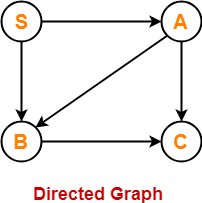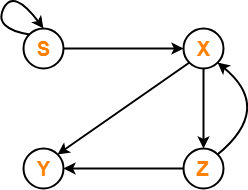## Context Free Language-

Before you go through this article, make sure that you have gone through the previous article on Context Free Language.

We have discussed-

• Context free language is generated using a context free grammar.
• Each context free language is accepted by a Pushdown automaton.

## Algorithm To Decide Whether CFL Is Finite Or Not-

For a given CFG, there exists an algorithm to decide whether its language is finite or not.

### Step-01:

Reduce the given grammar completely by-

• Eliminating ∈ productions
• Eliminating unit productions
• Eliminating useless productions

Also Watch- How To Reduce Grammar?

### Step-02:

• Draw a directed graph whose nodes are variables of the given grammar.
• There exists an edge from node A to node B if there exists a production of the form A → αBβ.

Now, following 2 cases are possible-

### Case-01:

• Directed graph contains a cycle.
• In this case, language of the given grammar is infinite.

### Case-02:

• Directed graph does not contain any cycle.
• In this case, language of the given grammar is finite.

Also Read- Algorithm To Decide Whether CFL Is Empty

## Problem-01:

Check whether language of the following grammar is finite or not-

S → AB / a

A → BC / b

B → CC / c

## Solution-

### Step-01:

The given grammar is already completely reduced.

### Step-02:

We will draw a directed graph whose nodes will be S , A , B , C.

Now,

• Due to the production S → AB, directed graph will have edges S → A and S → B.
• Due to the production A → BC, directed graph will have edges A → B and A → C.
• Due to the production B → CC, directed graph will have edge B → C.

The required directed graph is-Clearly,

• The directed graph does not contain any cycle.
• Therefore, language of the given grammar is finite.

## Problem-02:

Check whether language of the following grammar is finite or not-

S → XS / b

X → YZ

Y → ab

Z → XY

## Solution-

### Step-01:

The given grammar is already completely reduced.

### Step-02:

We will draw a directed graph whose nodes will be S , X , Y , Z.

Now,

• Due to the production S → XS / b, directed graph will have edges S → X and S → S.
• Due to the production X → YZ, directed graph will have edges X → Y and X → Z.
• Due to the production Z → XY, directed graph will have edges Z → X and Z → Y.

The required directed graph is-Clearly,

• The directed graph contain cycles.
• Therefore, language of the given grammar is infinite.

Next Article- CYK Algorithm

Get more notes and other study material of Theory of Automata and Computation.

Watch video lectures by visiting our YouTube channel LearnVidFun.

## Context Free Language-

Before you go through this article, make sure that you have gone through the previous article on Context Free Language.

We have discussed-

• Context free language is generated using a context free grammar.
• Each context free language is accepted by a Pushdown automaton.

## Algorithm To Decide Whether CFL Is Empty Or Not-

 If we can not derive any string of terminals from the given grammar,then its language is called as an Empty Language.L(G) = ϕ

For a given CFG, there exists an algorithm to decide whether its language is empty L(G) = ϕ or not.

### Algorithm-

• Remove all the useless symbols from the grammar.
• A useless symbol is one that does not derive any string of terminals.
• If the start symbol is found to be useless, then language is empty otherwise not.

### Remember

The language generated from a CFG is non-empty iff the start symbol is generating.

## Example-

Consider the following grammar-

S → XY

X → AX

X → AA

A → a

Y → BY

Y → BB

B → b

Now, let us check whether language generated by this grammar is empty or not.

The given grammar can be written as-

S → aabb

X → aX

X → aa

A → a

Y → bY

Y → bb

B → b

Clearly,

• The start symbol generates at least one string (many more are possible).
• Therefore, start symbol is useful.
• Thus, language generated by the given grammar is non-empty.

 L(G) ≠ ϕ

## NOTE-

If L(G) = { ∈ } i.e.

• If the language generated by a grammar contains only a null string,
• then it is considered as non-empty.

Next Article- Algorithm To Decide Whether CFL Is Finite

Get more notes and other study material of Theory of Automata and Computation.

Watch video lectures by visiting our YouTube channel LearnVidFun.